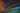# Property Tests - Testing the characteristics of your data rather than the data itself

Jordan PolaniecOct 2019
software-testingfscheck

We've all seen a standard unit test before. They are simple and ideally an elegant small snippet of code that tests a very tiny portion of the bigger picture.

``````[Test]
public void CurrencyFormatDisplayValueTest_Example() {
var ck = new Currency("Currency") {
Value = 123.46m,
CurrencyFormat = new CurrencyFormat {
CurrencySymbol = "\$",
DecimalPlaces = 2,
DecimalSymbol = "."
}
};
Assert.AreEqual("\$123.46", ck.DisplayValue); // en-US
}
``````

This test verifies that a decimal sent into a `Currency` object shows the proper `DisplayValue` when accessed. In this case, the 'DisplayValue`property determines what the client would render in the UI. We want to test that the`DisplayValue``` property is rendering data in the expected format. The```Value` property is the raw object value, uglified and shielded from the user's delicate eyes in the UI.

The idea behind this type of test - an Example Unit Test - is to have an easily repeatable way to verify an object's behavior. In the case above however, only one value is tested. Surely more than one value is possible for a consumer to enter. The assumption is made that this value covers enough of the scope of this functionality to not warrant using other values in additional tests.(By the way, code coverage for `Currency.get_DisplayValue()` now equals 100% despite only having our single test.)

What if we realize we need more tests to ensure this actually does what it says it does?

### TestCases with unit tests

Well, thanks to major test frameworks having support for parameterized tests, we can do this with relative ease:

``````[Test]
[TestCase(123.453, "\$123.45")]
[TestCase(52325.2341, "\$52,325.23")]
[TestCase(-32082.432, "(\$32,082.43)")]
public void CurrencyFormatDisplayValueTest_Example_TestCases(double value, string expectedDisplayValue) {
var ck = new Currency("Currency") {
Value = new decimal(value),
CurrencyFormat = new CurrencyFormat {
CurrencySymbol = "\$",
DecimalPlaces = 2,
DecimalSymbol = ".",
DigitGroupingSymbol = ",",
DigitsInGroup = 3,
}
};
Assert.AreEqual(expectedDisplayValue, ck.DisplayValue); // en-US
}
``````

Now multiple tests will be run and multiple values tested without the need to write the same test code more than once. However, this doesn't really cover any cases off the happy path, right? We need more test cases. Now our test looks like this:

``````[Test]
[TestCase(123.453, "\$123.45")]
[TestCase(52325.2341, "\$52,325.23")]
[TestCase(-32082.432, "(\$32,082.43)")]
[TestCase(0, "\$0.00")]
[TestCase(double.NaN, "\$0.00")]
public void CurrencyFormatDisplayValueTest_Example_MoreTestCases(double value, string expectedDisplayValue) {
var ck = new Currency("Currency") {
Value = new decimal(value),
CurrencyFormat = new CurrencyFormat {
CurrencySymbol = "\$",
DecimalPlaces = 2,
DecimalSymbol = "."
}
};
Assert.AreEqual(expectedDisplayValue, ck.DisplayValue); // en-US
}
``````

Our test is acquiring a decent amount of cases that we feel obligated to cover. And this is only one type of test on a single property. Just think of all the test cases we are going to have to keep track of if we want to have this same type of rigorous testing on multiple tests. Sure, test frameworks have features like `TestCaseSource` that allow you to pass in an enumerable of options to a test, but we're still manually maintaining all these potential cases. Is there a way to help cover all these options(and potentially more) without having to maintain them in our codebase?

Let's take a look at the code we're testing:

``````//In our Currency.cs:

///
/// String representation of the backing currency value. Utilizes CurrencyFormat to present a UI-friendly currency value.
///

public override string DisplayValue {
get {
if(!Value.Equals(default(decimal))) {
return GetRudimentaryDisplayValueFromCurrencyFormat(CurrencyFormat);
}

return "\$0.00";
}
}

//...Currency.GetRudimentaryDisplayValueFromCurrencyFormat

//Our(non-production) way of generating a display value from currency format options
private string GetRudimentaryDisplayValueFromCurrencyFormat(CurrencyFormat currencyFormat) {
if(Value < 0.0001m) { return "\$0.00"; }

//Value is the raw(object) value of the Currency.
string friendlyValue = Value.ToString();

//assign currency symbol
friendlyValue = currencyFormat.CurrencySymbol + friendlyValue;

//assign decimal symbol and decimal places

var decimalPointSplit = friendlyValue.Split(new [] { currencyFormat.DecimalSymbol }, StringSplitOptions.None);

friendlyValue = decimalPointSplit.First() + currencyFormat.DecimalSymbol + decimalPointSplit.Last().Substring(0, currencyFormat.DecimalPlaces);

return friendlyValue;
}
``````

When writing Property tests, we want to assert that the value under test has specific characteristics rather than that it is a specific value. Put more concisely, we want to test the "Properties" of the value.

### Example Property Test

In our example the `DisplayValue` rendered will have 4 main properties that we want to verify(it's definitely possible there are more we could assert on):

• A specific currency symbol at the front of the value
• A specific decimal symbol somewhere in the value
• Correct amount of the specified decimal symbol at the appropriate point in the Value
• The amount of decimal places is expected

These 4 characteristics will be present on every `DisplayValue`. Note how none of these properties pertain to specific values. When creating a Property test we care that these characteristics are present in the value under test. Here's our initial Property Tests, one for each of the characteristics we want to measure:

``````[Test]
[TestCase(123.456, "\$123.46", "\$", 2, ".")]
public void CurrencySymbolIsAtFront(double value, string expectedDisplayValue, string currencySymbol, int decimalPlaces, string decimalSymbol) {
var currency = new Currency("CurrencyTest") {
Value = new decimal(value),
CurrencyFormat = new CurrencyFormat { CurrencySymbol = currencySymbol, DecimalPlaces = decimalPlaces, DecimalSymbol = decimalSymbol }
};
string actualCurrencySymbol = currency.DisplayValue.ToString();
Assert.That(actualCurrencySymbol, Is.EqualTo(currency.CurrencyFormat.CurrencySymbol));
}

[Test]
[TestCase(123.456, "\$123.46", "\$", 2, ".")]
public void CurrencySymbolIsPresent(double value, string expectedDisplayValue, string currencySymbol, int decimalPlaces, string decimalSymbol) {

var currency = new Currency("CurrencyTest") {
Value = new decimal(value),
CurrencyFormat = new CurrencyFormat {
CurrencySymbol = currencySymbol,
DecimalPlaces = decimalPlaces,
DecimalSymbol = decimalSymbol
}
};
Assert.That(currency.DisplayValue.Contains(decimalSymbol));
}

[Test]
[TestCase(123.456, "\$123.46", "\$", 2, ".")]
public void CorrectAmountOfDecimalSymbolsArePresent(double value, string expectedDisplayValue, string currencySymbol, int decimalPlaces, string decimalSymbol) {
var currency = new Currency("CurrencyTest") {
Value = new decimal(value),
CurrencyFormat = new CurrencyFormat {
CurrencySymbol = currencySymbol,
DecimalPlaces = decimalPlaces,
DecimalSymbol = decimalSymbol
}
};

//assert decimal symbol is present
Assert.That(currency.DisplayValue.Contains(decimalSymbol));

//assert the correct amount of decimal symbols are present

var matches = Regex.Matches(currency.DisplayValue, \$"[{decimalSymbol}]");
Assert.That(matches.Count, Is.EqualTo(1));
}

[Test]
[TestCase(123.456, "\$123.46", "\$", 2, ".")]
public void CorrectAmountOfDecimalPlacesArePresent(double value, string expectedDisplayValue, string currencySymbol, int decimalPlaces, string decimalSymbol) {
var currency = new Currency("CurrencyTest") {
Value = new decimal(value),
CurrencyFormat = new CurrencyFormat {
CurrencySymbol = currencySymbol,
DecimalPlaces = decimalPlaces,
DecimalSymbol = decimalSymbol
}
};

//get value after decimal symbol

string afterDecimalSymbol = currency.DisplayValue.Split(new [] { currency.CurrencyFormat.DecimalSymbol }, StringSplitOptions.None).Last();
Assert.That(afterDecimalSymbol.Length, Is.EqualTo(currency.CurrencyFormat.DecimalPlaces));
}
``````

Notice how we're never asserting on the actual value in any way here, just that the value has the properties of what we have defined to be a valid, good `DisplayValue`. There's one glaring issue though. Look at the amount of `TestCase` parameters we have. 5 parameters for this test. If we want more test cases that's 5 more parameters per test case! It seems like things just got more complicated to maintain than before. We could wrap them into an object and use `TestCaseSource`, but in that case we still need to come up with all these individual test cases ourselves. That's where tools like FsCheck come in.

### FsCheck

FsCheck is the .NET flavor of QuickCheck of Haskell Property testing fame(it also borrows from ScalaCheck). This seems to be the premier .NET Property testing framework. FsCheck's roots are in F#, but C# support is robust as well thanks to .NET. FsCheck's main goal is to help you generate these test cases, based on criteria you, the test writer, specify. There are NUnit and xUnit extensions so that these generated values slide into test suites and take advantage of unit test result frameworks. We'll be using FsCheck's NUnit integration in our example. Let's take a look at one of the above tests refactored to use FsCheck for generating test cases.

``````[Test]
public void CurrencySymbolIsAtFront_FsCheck() {
Prop.ForAll(CurrencyFormats(), CurrencyValues(),(format, value) => {
var currency = new Currency("CurrencyTest", format, value);

//Write out the current test data for this exercise.
Console.WriteLine(\$"Value = {currency.Value} | " +
\$"DisplayValue = {currency.DisplayValue} | " +
\$"Decimal Symbol = {format.DecimalSymbol} | " +
\$"Decimal Places = {format.DecimalPlaces} | " +
\$"Currency Symbol = {format.CurrencySymbol}");

string actualCurrencySymbol = currency.DisplayValue.ToString();

Assert.That(actualCurrencySymbol, Is.EqualTo(currency.CurrencyFormat.CurrencySymbol));
}).QuickCheckThrowOnFailure();
}
``````

First off, notice how our test is inside this `Prop.ForAll` method. This is an FsCheck construct that allows consumers to pass in delegates that generate values and act on those values. Each of these sent in pieces of data is part of what we will base our assertions on to determine a passing or failing test. The `QuickCheckThrowOnFailure()` call at the end is part of the NUnit extension and forwards results to the test runner so they can be reported upon. There are various methods to choose from here depending on what you want to output.

Second, take a look at the `CurrencyFormats` and `CurrencyValues` methods. These methods are where we generate the value used in the test. More specifically, they are the specs to which FsCheck operates.

``````//CurrencyValues()

private static Arbitrary CurrencyValues() => Arb.Generate().Where(d => d <= 99999999 && d > 0).ToArbitrary();
``````

`Arb.Generate` creates another FsCheck construct of a specific type. `Where()` is fairly self-descriptive in this case. We want to keep the decimal that is generated between a specific range.

What's the `Arbitrary` for? Go back to the `Prop.ForAll()` call in the actual test. One of `Prop.ForAll`'s overloads is `Prop.ForAll(Arbitrary arb1, Arbitrary arb2, Action body)`. When executing `ForAll`, FsCheck will internally use these `Arbitrary` instances to keep track of what worked and what didn't work and use that to tailor its future generated test cases. `Arbitrary` is the Type in which data generation is performed in FsCheck. There are many ways to customize how this does generation so it's important to get familiar with all it can be told to do.

This plays into the concept of Shrinking in Property tests. When a failing case is found while running, FsCheck will attempt to find the minimum input that causes the assertion to no longer hold true on the property. This is the "shrunk" input.

Let's take a look at the `CurrencyFormats()` spec - something a bit more complex:

``````//CurrencyFormats()
private static Arbitrary CurrencyFormats() {
Gen possibleCurrencySymbols = Gen.Elements("\$", "¥");
Gen possibleDecimalSymbols = Gen.Elements(",", ".");
Gen possibleDecimalPlaces = Gen.Choose(1, 10);

Gen genCurrencyFormat = from dig in Arb.Generate().Where(i => i <= 3 && i > 0)
from currencySymbol in possibleCurrencySymbols
from decimalSymbol in possibleDecimalSymbols
from decimalPlaces in possibleDecimalPlaces
select new CurrencyFormat {
CurrencySymbol = currencySymbol,
DecimalSymbol = decimalSymbol,
DecimalPlaces = decimalPlaces,
};

return genCurrencyFormat.ToArbitrary();
}
``````

Digging in, FsCheck's `Gen.Elements` call lets the consumer specify a list of values they want as possibilities for selection. `Gen.Choose` allows the consumer to specify a range of integers for selection. We take advantage of these specs in the linq statements right below `Gen.Choose`. The result is a `Gen` of type `CurrencyFormat`. This is essentially our spec to generate test cases. We then convert it to an `Arbitary` so that we can generate(you guessed it) arbitrary `CurrencyFormat` instances to get the `DisplayValue`s of and assert on their 4 main properties.

By default, FsCheck will generate 100 test cases from the `Gen` spec. Each of these will be fed through our `Prop.ForAll` call and your unit test assertions performed on them.

Our example calls `QuickCheckThrowOnFailure` because we want to fail the test in nunit when it comes across one that fails our assertions.

Speaking of failures, let's run our unit tests now..

What does it look like when a fail case is found? FsCheck has found an issue while executing test cases. Let's see what it came across when running our above `CurrencySymbolIsAtFront_FsCheck` test: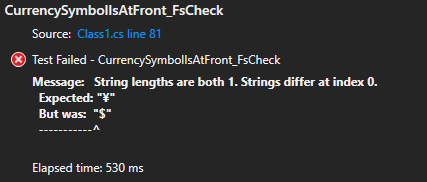It looks like the following case produced an issue based on Console output we setup in our test:

``````(Test Data)
Value = 0.00000147573952568201576453 | DisplayValue = \$0.0000000 | Decimal Symbol = . | Decimal Places = 10 | Currency Symbol = ¥
``````

Looking at our rudimentary DisplayValue generation code we have an issue. We're catching decimal values less than 0.0001 and returning a "\$" every time regardless of what the specified currency format is. That should be a quick fix:

``````//inside our rudimentary displayvalue forming method...

if (Value < 0.0001m) { return currencyFormat.CurrencySymbol + "0.00"; }
``````

The test now passes. Let's refactor another test to use FsCheck using the same template we applied to the previous one:

``````[Test]
public void CurrencySymbolIsPresent_FsCheck() { Prop.ForAll(CurrencyFormats(), CurrencyValues(), (format, value) =>
{
var currency = new Currency("CurrencyTest", format, value);

//Write out the current test data for this exercise.
Console.WriteLine (\$"Value = {currency.Value} | " +
\$"DisplayValue = {currency.DisplayValue} | " +
\$"Decimal Symbol = {format.DecimalSymbol} | " +
\$"Decimal Places = {format.DecimalPlaces} | " +
\$"Currency Symbol = {format.CurrencySymbol}");

//assert decimal symbol is present

}).QuickCheckThrowOnFailure ();
}
``````

Running this unit test produces another failure. This time, when asserting the DisplayValue has the correct decimal symbol FsCheck found a failing case.

``````(Test Data)
Value = 0.0000055340232307028000752 | DisplayValue = ¥0.00 | Decimal Symbol = , | Decimal Places = 9 | Currency Symbol = ¥
``````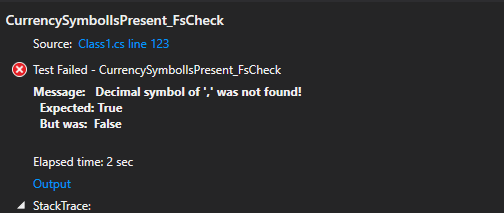Taking another look at our code we see a "." is being hardcoded into values less than 0.0001. Remember in our spec for generating CurrencyFormats the decimal symbol may not always be a ".".

``````if(Value < 0.0001m) {
return currencyFormat.CurrencySymbol + "0.00";
}
``````

That should be fixed:

``````//fixed
if(Value < 0.0001m) { return currencyFormat.CurrencySymbol + "0" + currencyFormat.DecimalSymbol + "00"; }
``````

Alright, this test now passes as well. Moving on, let's refactor another of our Property tests to use FsCheck:

``````[Test]
public void CorrectAmountOfDecimalSymbolsArePresent_FsCheck() {
Prop.ForAll(CurrencyFormats(), CurrencyValues(),(format, value) => {
var currency = new Currency("CurrencyTest", format, value);

//assert decimal symbol is present
Assert.That(currency.DisplayValue.Contains(format.DecimalSymbol));

//assert the correct amount of decimal symbols are present
var matches = Regex.Matches(currency.DisplayValue, \$"[{format.DecimalSymbol}]");

Assert.That(matches.Count, Is.EqualTo(1));

}).QuickCheckThrowOnFailure();
}
``````

This test passes. Great! Let's modify the last one to use FsCheck:

``````[Test]
public void CorrectAmountOfDecimalPlacesArePresent_FsCheck() {
Prop.ForAll(CurrencyFormats(), CurrencyValues(),(format, value) => {
var currency = new Currency("CurrencyTest", format, value);

//Write out the current test data for this exercise.
Console.WriteLine(\$"Value = {currency.Value} | " +
\$"DisplayValue = {currency.DisplayValue} | " +
\$"Decimal Symbol = {format.DecimalSymbol} | " +
\$"Decimal Places = {format.DecimalPlaces} | " +
\$"Currency Symbol = {format.CurrencySymbol}");

//get value after decimal symbol
string afterDecimalSymbol =
currency.DisplayValue.Split(new [] { currency.CurrencyFormat.DecimalSymbol }, StringSplitOptions.None)
.Last();

Assert.That(afterDecimalSymbol.Length, Is.EqualTo(currency.CurrencyFormat.DecimalPlaces),
\$"Incorrect number of decimal places in display value.");

}).QuickCheckThrowOnFailure();
}
``````

Looks like FsCheck found another issue when we run this test:

``````(Test Data)
Value = 0.0000000036893488156009037823 | DisplayValue = ¥0.00 | Decimal Symbol = . | Decimal Places = 10 | Currency Symbol = ¥
``````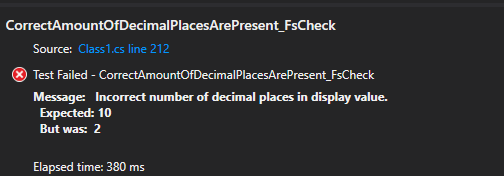This time we were expecting 10 decimal places to be on the returned DisplayValue when the value is less than 0.0001, but only 2 were present.

Let's go ahead and fix that issue in our code:

``````//the resulting(still non-production) method for creating our DisplayValue
private string GetRudimentaryDisplayValueFromCurrencyFormat(CurrencyFormat currencyFormat) {
string friendlyValue = string.Empty;

if(Value < 0.0001m) {
friendlyValue = currencyFormat.CurrencySymbol + "0" + currencyFormat.DecimalSymbol;

return friendlyValue;
}

//Value is the raw(object) value of the Currency.
friendlyValue = Value.ToString();

//assign currency symbol
friendlyValue = currencyFormat.CurrencySymbol + friendlyValue;

//assign decimal symbol and decimal places
var decimalPointSplit = friendlyValue.Split(new [] { currencyFormat.DecimalSymbol }, StringSplitOptions.None);
friendlyValue = decimalPointSplit.First() + currencyFormat.DecimalSymbol + decimalPointSplit.Last().Substring(0, currencyFormat.DecimalPlaces);

return friendlyValue;
}
``````

Now we're padding the amount of decimal places specified on the currencyFormat.

Ok, we've refactored our Property tests to all use FsCheck. Running through all 4 again… we can see that FsCheck found no more errors.

Here's a sample of the generated test cases from our Console output in the test: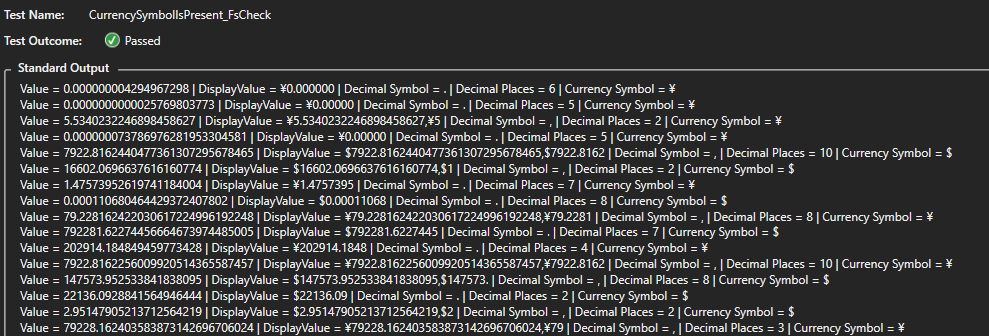…(more results omitted for brevity)

And at the very bottom of the results…

Ok, passed 100 tests.

We can see there was a vast array of test cases generated and we never had to manually specify any of these parameters. Keep in mind you can still see this output when the test fails.

At the bottom of our generated test case output we can see FsCheck has written, "Ok, passed 100 tests." Remember, you can modify how many test cases are generated if you want. 100 is the default amount.

During this small refactoring exercise in testing our method for generating a DisplayValue FsCheck helped us find several issues that we were able to fix. While finding those issues we didn't have to think of specific test cases by ourselves, FsCheck did that for us. We just specified the boundaries of what should be sent in as test data.

In our example unit test we performed several assertions in one test. In order maintain clarity we could break these up into their own tests so when something fails we get a bit more granularity in the results.

Property testing is not the solution to every unit test and Example-based unit tests definitely fulfill many of the requirements of testing. However, Property testing can be very useful when a potentially large amount of input can be passed or data is highly variable(and output reflects this). When output will contain memorable characteristics in this you may want to consider Property testing.